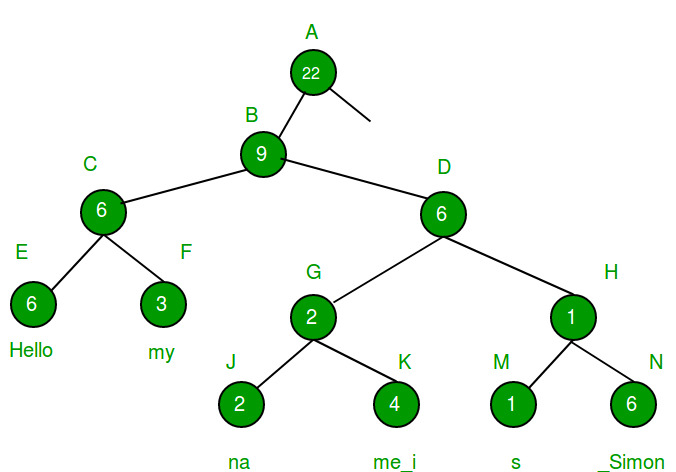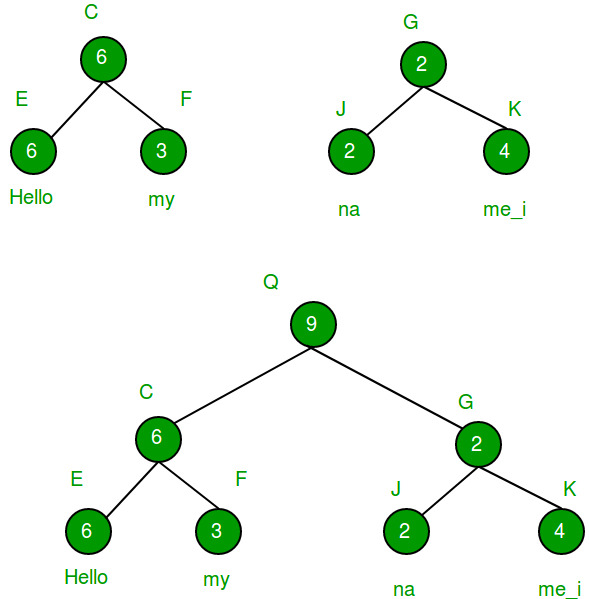# Ropes Data Structure (Fast String Concatenation)

• Difficulty Level : Hard
• Last Updated : 20 Jul, 2022

One of the most common operations on strings is appending or concatenation. Appending to the end of a string when the string is stored in the traditional manner (i.e. an array of characters) would take a minimum of O(n) time (where n is the length of the original string).
We can reduce time taken by append using Ropes Data Structure.

Ropes Data Structure

A Rope is a binary tree structure where each node except the leaf nodes, contains the number of characters present to the left of that node. Leaf nodes contain the actual string broken into substrings (size of these substrings can be decided by the user).
Consider the image below.The image shows how the string is stored in memory. Each leaf node contains substrings of the original string and all other nodes contain the number of characters present to the left of that node. The idea behind storing the number of characters to the left is to minimise the cost of finding the character present at i-th position.
1. Ropes drastically cut down the cost of appending two strings.
2. Unlike arrays, ropes do not require large contiguous memory allocations.
3. Ropes do not require O(n) additional memory to perform operations like insertion/deletion/searching.
4. In case a user wants to undo the last concatenation made, he can do so in O(1) time by just removing the root node of the tree.
1. The complexity of source code increases.
2. Greater chances of bugs.
3. Extra memory required to store parent nodes.
4. Time to access i-th character increases.
Now let’s look at a situation that explains why Ropes are a good substitute to monolithic string arrays.
Given two strings a[] and b[]. Concatenate them in a third string c[].
Examples:

```Input  : a[] = "This is ", b[] = "an apple"
Output : "This is an apple"

Input  : a[] = "This is ", b[] = "geeksforgeeks"
Output : "This is geeksforgeeks"```

Method 1 (Naive method)

We create a string c[] to store concatenated string. We first traverse a[] and copy all characters of a[] to c[]. Then we copy all characters of b[] to c[].
Implementation:

## C++

 `// Simple C++ program to concatenate two strings``#include ``using` `namespace` `std;` `// Function that concatenates strings a[0..n1-1]``// and b[0..n2-1] and stores the result in c[]``void` `concatenate(``char` `a[], ``char` `b[], ``char` `c[],``                              ``int` `n1, ``int` `n2)``{``    ``// Copy characters of A[] to C[]``    ``int` `i;``    ``for` `(i=0; i

## Java

 `//Java program to concatenate two strings` `class` `GFG {` `    ``// Function that concatenates strings a[0..n1-1]``    ``// and b[0..n2-1] and stores the result in c[]``    ``static` `void` `concatenate(``char` `a[], ``char` `b[], ``char` `c[],``            ``int` `n1, ``int` `n2) {``        ``// Copy characters of A[] to C[]``        ``int` `i;``        ``for` `(i = ``0``; i < n1; i++) {``            ``c[i] = a[i];``        ``}` `        ``// Copy characters of B[]``        ``for` `(``int` `j = ``0``; j < n2; j++) {``            ``c[i++] = b[j];``        ``}` `    ``}` `    ``// Driver code``    ``public` `static` `void` `main(String[] args) {``        ``char` `a[] = ``"Hi This is geeksforgeeks. "``.toCharArray();``        ``int` `n1 = a.length;` `        ``char` `b[] = ``"You are welcome here."``.toCharArray();``        ``int` `n2 = b.length;` `        ``// Concatenate a[] and b[] and store result``        ``// in c[]``        ``char` `c[] = ``new` `char``[n1 + n2];``        ``concatenate(a, b, c, n1, n2);``        ``for` `(``int` `i = ``0``; i < n1 + n2 - ``1``; i++) {``            ``System.out.print(c[i]);``        ``}` `    ``}``}``// This code is contributed by PrinciRaj1992`

## Python3

 `# Python3 program to concatenate two strings` `# Function that concatenates strings a[0..n1-1]``# and b[0..n2-1] and stores the result in c[]``def` `concatenate(a, b, c, n1, n2):` `    ``# Copy characters of A[] to C[]``    ``i ``=` `-``1``    ``for` `i ``in` `range``(n1):``        ``c[i] ``=` `a[i]` `    ``# Copy characters of B[]``    ``for` `j ``in` `range``(n2):``        ``c[i] ``=` `b[j]``        ``i ``+``=` `1` `# Driver Code``if` `__name__ ``=``=` `"__main__"``:``    ``a ``=` `"Hi This is geeksforgeeks. "``    ``n1 ``=` `len``(a)` `    ``b ``=` `"You are welcome here."``    ``n2 ``=` `len``(b)` `    ``a ``=` `list``(a)``    ``b ``=` `list``(b)` `    ``# Concatenate a[] and b[] and``    ``# store result in c[]``    ``c ``=` `[``0``] ``*` `(n1 ``+` `n2 ``-` `1``)` `    ``concatenate(a, b, c, n1, n2)` `    ``for` `i ``in` `c:``        ``print``(i, end ``=` `"")` `# This code is contributed by``# sanjeev2552`

## C#

 `// C# program to concatenate two strings``using` `System;` `public` `class` `GFG {`` ` `    ``// Function that concatenates strings a[0..n1-1]``    ``// and b[0..n2-1] and stores the result in c[]``    ``static` `void` `concatenate(``char` `[]a, ``char` `[]b, ``char` `[]c,``            ``int` `n1, ``int` `n2) {``        ``// Copy characters of A[] to C[]``        ``int` `i;``        ``for` `(i = 0; i < n1; i++) {``            ``c[i] = a[i];``        ``}`` ` `        ``// Copy characters of B[]``        ``for` `(``int` `j = 0; j < n2; j++) {``            ``c[i++] = b[j];``        ``}`` ` `    ``}`` ` `    ``// Driver code``    ``public` `static` `void` `Main() {``        ``char` `[]a = ``"Hi This is geeksforgeeks. "``.ToCharArray();``        ``int` `n1 = a.Length;`` ` `        ``char` `[]b = ``"You are welcome here."``.ToCharArray();``        ``int` `n2 = b.Length;`` ` `        ``// Concatenate a[] and b[] and store result``        ``// in c[]``        ``char` `[]c = ``new` `char``[n1 + n2];``        ``concatenate(a, b, c, n1, n2);``        ``for` `(``int` `i = 0; i < n1 + n2 - 1; i++) {``            ``Console.Write(c[i]);``        ``}`` ` `    ``}``}``/*This code is contributed by PrinciRaj1992*/`

Output:

`Hi This is geeksforgeeks. You are welcome here`

Time complexity : O(n)
Now let’s try to solve the same problem using Ropes.

Method 2 (Rope structure method)

This rope structure can be utilized to concatenate two strings in constant time.
1. Create a new root node (that stores the root of the new concatenated string)
2. Mark the left child of this node, the root of the string that appears first.
3. Mark the right child of this node, the root of the string that appears second.
And that’s it. Since this method only requires to make a new node, it’s complexity is O(1).
Consider the image below (Image source : https://en.wikipedia.org/wiki/Rope_(data_structure))Implementation:

## CPP

 `// C++ program to concatenate two strings using``// rope data structure.``#include ``using` `namespace` `std;` `// Maximum no. of characters to be put in leaf nodes``const` `int` `LEAF_LEN = 2;` `// Rope structure``class` `Rope``{``public``:``    ``Rope *left, *right, *parent;``    ``char` `*str;``    ``int` `lCount;``};` `// Function that creates a Rope structure.``// node --> Reference to pointer of current root node``//   l  --> Left index of current substring (initially 0)``//   r  --> Right index of current substring (initially n-1)``//   par --> Parent of current node (Initially NULL)``void` `createRopeStructure(Rope *&node, Rope *par,``                         ``char` `a[], ``int` `l, ``int` `r)``{``    ``Rope *tmp = ``new` `Rope();``    ``tmp->left = tmp->right = NULL;`` ` `    ``// We put half nodes in left subtree``    ``tmp->parent = par;`` ` `    ``// If string length is more``    ``if` `((r-l) > LEAF_LEN)``    ``{``        ``tmp->str = NULL;``        ``tmp->lCount = (r-l)/2;``        ``node = tmp;``        ``int` `m = (l + r)/2;``        ``createRopeStructure(node->left, node, a, l, m);``        ``createRopeStructure(node->right, node, a, m+1, r);``    ``}``    ``else``    ``{``        ``node = tmp;``        ``tmp->lCount = (r-l);``        ``int` `j = 0;``        ``tmp->str = ``new` `char``[LEAF_LEN];``        ``for` `(``int` `i=l; i<=r; i++)``            ``tmp->str[j++] = a[i];``    ``}``}` `// Function that prints the string (leaf nodes)``void` `printstring(Rope *r)``{``    ``if` `(r==NULL)``        ``return``;``    ``if` `(r->left==NULL && r->right==NULL)``        ``cout << r->str;``    ``printstring(r->left);``    ``printstring(r->right);``}` `// Function that efficiently concatenates two strings``// with roots root1 and root2 respectively. n1 is size of``// string represented by root1.``// root3 is going to store root of concatenated Rope.``void` `concatenate(Rope *&root3, Rope *root1, Rope *root2, ``int` `n1)``{``    ``// Create a new Rope node, and make root1``    ``// and root2 as children of tmp.``    ``Rope *tmp = ``new` `Rope();``    ``tmp->parent = NULL;``    ``tmp->left = root1;``    ``tmp->right = root2;``    ``root1->parent = root2->parent = tmp;``    ``tmp->lCount = n1;` `    ``// Make string of tmp empty and update``    ``// reference r``    ``tmp->str = NULL;``    ``root3 = tmp;``}` `// Driver code``int` `main()``{``    ``// Create a Rope tree for first string``    ``Rope *root1 = NULL;``    ``char` `a[] =  ``"Hi This is geeksforgeeks. "``;``    ``int` `n1 = ``sizeof``(a)/``sizeof``(a);``    ``createRopeStructure(root1, NULL, a, 0, n1-1);` `    ``// Create a Rope tree for second string``    ``Rope *root2 = NULL;``    ``char` `b[] =  ``"You are welcome here."``;``    ``int` `n2 = ``sizeof``(b)/``sizeof``(b);``    ``createRopeStructure(root2, NULL, b, 0, n2-1);` `    ``// Concatenate the two strings in root3.``    ``Rope *root3 = NULL;``    ``concatenate(root3, root1, root2, n1);` `    ``// Print the new concatenated string``    ``printstring(root3);``    ``cout << endl;``    ``return` `0;``}`

Output:

`Hi This is geeksforgeeks. You are welcome here.`

Time Complexity: O(1)

Auxiliary Space: O(1)
This article is contributed by Akhil Goel. If you like GeeksforGeeks and would like to contribute, you can also write an article using write.geeksforgeeks.org or mail your article to review-team@geeksforgeeks.org. See your article appearing on the GeeksforGeeks main page and help other Geeks.
Please write comments if you find anything incorrect, or you want to share more information about the topic discussed above.

My Personal Notes arrow_drop_up